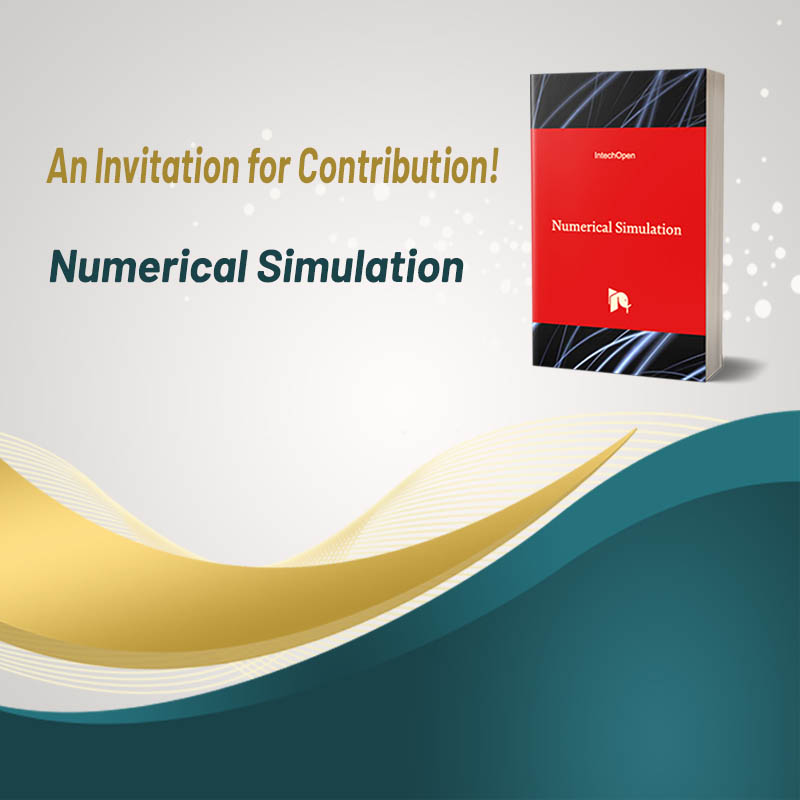# Numerical Simulation

An Invitation for Contribution!

Numerical SimulationA numerical simulation is a calculation that is run on a computer following a program that implements a mathematical model for a physical system. Numerical simulations are required to study the behavior of systems whose mathematical models are too complex to provide analytical solutions, as in most nonlinear systems. This book aimed to cover different computational techniques for simulating a large variety of engineering and physical systems. Applications are drawn from aerospace, mechanical, electrical, chemical, biological, and mining engineering. Topics include mathematical formulations; network problems; sparse direct and iterative matrix solution techniques; Newton methods for nonlinear problems; discretization methods for ordinary, time-periodic, and partial differential equations; fast methods for partial differential and integral equations; techniques for dynamical system model reduction, approaches for molecular dynamics and Monte Carlo techniques. This book will interest researchers, scientists, engineers, and graduate students in many disciplines, who use mathematical modeling and computer simulation. The book will undoubtedly serve as a valuable tool for researchers interested in getting involved in this multidisciplinary field. Furthermore, it will be helpful to encourage further experimental and theoretical research in the areas mentioned above of numerical simulation.

A numerical simulation is a calculation that is run on a computer following a program that implements a mathematical model for a physical system. Numerical simulations are required to study the behavior of systems whose mathematical models are too complex to provide analytical solutions, as in most nonlinear systems. This book aimed to cover different computational techniques for simulating a large variety of engineering and physical systems. Applications are drawn from aerospace, mechanical, electrical, chemical, biological, and mining engineering. Topics include mathematical formulations; network problems; sparse direct and iterative matrix solution techniques; Newton methods for nonlinear problems; discretization methods for ordinary, time-periodic, and partial differential equations; fast methods for partial differential and integral equations; techniques for dynamical system model reduction, approaches for molecular dynamics and Monte Carlo techniques. This book will interest researchers, scientists, engineers, and graduate students in many disciplines, who use mathematical modeling and computer simulation. The book will undoubtedly serve as a valuable tool for researchers interested in getting involved in this multidisciplinary field. Furthermore, it will be helpful to encourage further experimental and theoretical research in the areas mentioned above of numerical simulation.

### Topics

The following topics illustrate the target subject areas and scope of the project. These keywords are not definitive but can be used as the basis for the chapter content. We accept theoretical and applied scientific papers which can be presented as original research papers and review papers. The required length of the full chapters is 10-20 pages.